{{announcement.body}}
{{announcement.title}}

# Improving C# Code Using Refactoring and Unit Testing

DZone 's Guide to

# Improving C# Code Using Refactoring and Unit Testing

### If you're hoping to improve your code quality, learn to use several refactoring and unit testing methods to turn bad code into beautiful code with these steps.

· DevOps Zone ·
Free Resource

Comment (2)

Save
{{ articles.views | formatCount}} Views

In this article, I want to introduce how to use refactoring and unit test in improving code quality through an example. My example is the Windows form application, whose main form looks like this:My simple application is used to calculate average mark of a student. The calculation is based on the student's math mark and literal mark and it varies according to the student’s area (country or city). Here are the formulas:

• Country: Average = (Math mark + Literal mark + 1) / 2

• City: Average = (Math mark + Literal mark) / 2

My C# code improving process is implemented with the steps shown in the figure below: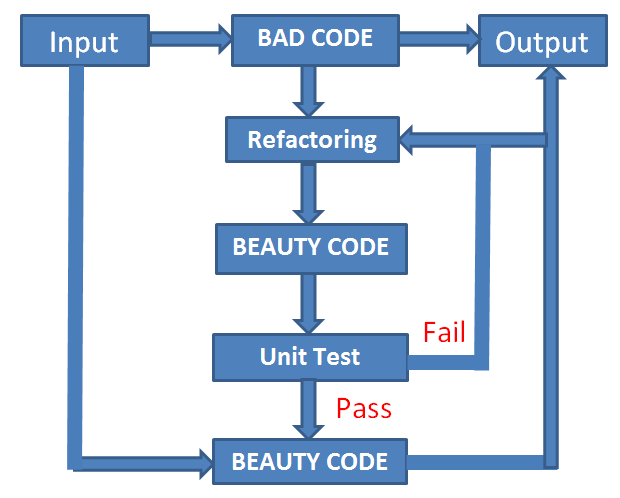## My C# Code Improving Process

`````` private void btnCalculate_Click(object sender, EventArgs e)
{
double result;
if (!double.TryParse(txtMath.Text, out result) )
{
MessageBox.Show("Error. Please enter a numeric value!");
txtMath.Select();
return;
}
if( ((Convert.ToDouble(txtMath.Text) > 10))
|| (Convert.ToDouble(txtMath.Text) < 0))
{
MessageBox.Show("Error. Please enter from 0 to 10!");
txtMath.Select();
return;
}
if (!double.TryParse(txtLiteral.Text, out result))
{
MessageBox.Show("Error. Please enter a numeric value!");
txtMath.Select();
return;
}
if (((Convert.ToDouble(txtLiteral.Text) > 10))
|| (Convert.ToDouble(txtMath.Text) < 0))
{
MessageBox.Show("Error. Please enter from 0 to 10!");
txtMath.Select();
return;
}
if (rbtnCountry.Checked)
{
txtAverage.Text = txtID.Text + " " + txtName.Text+ "  "
+ ((Convert.ToDouble(txtMath.Text)
+ Convert.ToDouble(txtLiteral.Text) + 1) / 2).ToString();
}

else {

txtAverage.Text = txtID.Text + " " + txtName.Text+ "  "
+ ((Convert.ToDouble(txtMath.Text)
+ Convert.ToDouble(txtLiteral.Text)) / 2).ToString();
}
}``````

### Refactoring

Decomposing the btnCalculate_Click method (extract method refactoring):

``````// Validate the Math mark input
private bool MathInputValid(string sMath)
{
double result;
if (!double.TryParse(sMath, out result))
{
MessageBox.Show("Error. Please enter a numeric value!");
return false;
}
if (((Convert.ToDouble(sMath) > 10)) || (Convert.ToDouble(sMath) < 0))
{
MessageBox.Show("Error. Please enter from 0 to 10!");
return false;
}
return true;
}
// Validate the Literal mark input
private bool LiteralInputValid(string sLiteral)
{
double result;
if (!double.TryParse(sLiteral, out result))
{
MessageBox.Show("Error. Please enter a numeric value!");
return false;
}
if (((Convert.ToDouble(sLiteral) > 10)) || (Convert.ToDouble(sLiteral) < 0))
{
MessageBox.Show("Error. Please enter from 0 to 10!");
return false;
}
return true;
}
// Calculation for students who live in country
private double CountryAvg(double dbMath, double dbLiteral)
{
return (dbMath + dbLiteral + 1) / 2;
}
// Calculation for students who live in city
private double CityAvg(double dbMath, double dbLiteral)
{
return (dbMath + dbLiteral) / 2;
}``````

So far, the btnCalculate_Click method looks like:

``````private void btnCalculate_Click(object sender, EventArgs e)
{
if(!MathInputValid(txtMath.Text))
{
txtMath.Select();
return;
}
if(!LiteralInputValid(txtLiteral.Text))
{
txtLiteral.Select();
return;
}
if (rbtnCountry.Checked)
{
txtAverage.Text = txtID.Text + " " + txtName.Text + "  "
+ CountryAvg(Convert.ToDouble(txtMath.Text),
Convert.ToDouble(txtLiteral.Text)).ToString();
}
else {
txtAverage.Text = txtID.Text + " " + txtName.Text+ "  "
+ CityAvg(Convert.ToDouble(txtMath.Text),
Convert.ToDouble(txtLiteral.Text)).ToString();
}
}``````

Add a Student class (extract class refactoring):

``````class Student
{
// fields
private string id;
private string name;
private double math;
private double literal;
// properties
public string ID
{
get { return id; }
set { id = value; }
}
public string NAME
{
get { return name; }
set { name = value; }
}
public double MATH
{
get { return math; }
set { math = value; }
}
public double LITERAL
{
get { return literal; }
set { literal = value; }
}

}``````

Moving the CountryAvg and the CityAvg methods to the Student class (move method refactoring):

``````private double CountryAvg(double dbMath, double dbLiteral)
{
return (dbMath + dbLiteral + 1) / 2;
}``````

The above is transformed into the following:

``````public double CountryAvg()
{
return (MATH + LITERAL + 1) / 2;
}``````
``````private double CityAvg(double dbMath, double dbLiteral)
{
return (dbMath + dbLiteral) / 2;
}``````

Then transformed into the following:

``````public double CityAvg()
{
return (MATH + LITERAL) / 2;
}``````

Creating the AREA Enum:

``````public enum AREA
{
Country,
City
}``````

Adding area field and Area property:

``````private AREA area;// field
public AREA Area // property
{
get { return area; }
set { area = value; }
}``````

Encapsulating CountryAvg and CityAvg():

``````public double AvgCalculate()
{
if (this.Area == AREA.Country)
{
return CountryAvg();
}
else
{
return CityAvg();
}
}``````

Making encapsulated methods private:

``````private double CityAvg()
private double CountryAvg()``````

The btnCalculate_Click() after creating the new Student class:

``````private void btnCalculate_Click(object sender, EventArgs e)
{
if(!MathInputValid(txtMath.Text))
{
txtMath.Select();
return;
}
if(!LiteralInputValid(txtLiteral.Text))
{
txtLiteral.Select();
return;
}
Student st = new Student();
if (rbtnCountry.Checked)
{
st.Area = AREA.Country;
}
else
{
st.Area = AREA.City;
}
st.ID = txtID.Text;
st.NAME = txtName.Text;
st.MATH = Convert.ToDouble(txtMath.Text);
st.LITERAL = Convert.ToDouble(txtLiteral.Text);
txtAverage.Text = st.ID + " " + st.NAME + " "
+ st.AvgCalculate().ToString();
}

``````

### Creating the Student Class Hierarchy

• Add two new classes that inherit the Student class: CountryStudent and CityStudent.

``````public class CityStudent: Student
public class CountryStudent: Student``````
• Move the CityAvg() method to the CityStudent class, and the CountryAvg() method to the CountryStudent class (pull-down method refactoring).

``````public  class CountryStudent: Student
{
public override double AvgCalculate()
{
return (MATH + LITERAL + 1) / 2;
}
}
public class CityStudent: Student
{
public override double AvgCalculate()
{
return (MATH + LITERAL) / 2;
}
}``````
• Since only some of the Student class members are implemented, this class is abstract and must be marked by the abstract keyword:

``public abstract class Student``
•  Methods in the Student class that are implemented in the CountryStudent class and the CityStudent class need to be marked as abstract. The AvgCalculate method in the Student class now looks like this:

``public abstract double AvgCalculate()``

### Beautiful Code

My bad code, after refactoring, looks like this:

``````public abstract class Student
{
// fields
private string id;
private string name;
private double math;
private double literal;
private AREA area;
// properties
public string ID
{
get { return id;
set { id = value; }
}
public string NAME
{
get { return name; }
set { name = value; }
}
public double MATH
{
get { return math; }
set { math = value; }
}
public double LITERAL
{
get { return literal; }
set { literal = value; }
}
public AREA Area
{
get { return area; }
set { area = value; }
}
// methods
public abstract double AvgCalculate();
}
public  class CountryStudent: Student
{
public override double AvgCalculate()
{
return (MATH + LITERAL + 1) / 2;
}
}
public class CityStudent: Student
{
public override double AvgCalculate()
{
return (MATH + LITERAL) / 2;
}
}
private void btnCalculate_Click(object sender, EventArgs e)
{
if(!MathInputValid(txtMath.Text))
{
txtMath.Select();
return;
}
if(!LiteralInputValid(txtLiteral.Text))
{
txtLiteral.Select();
return;
}
Student st;
if (rbtnCountry.Checked)
{
st = new CountryStudent();
}
else
{
st = new CityStudent();
}
st.ID = txtID.Text;
st.NAME = txtName.Text;
st.MATH = Convert.ToDouble(txtMath.Text);
st.LITERAL = Convert.ToDouble(txtLiteral.Text);
txtAverage.Text = st.ID + " " + st.NAME + " " + st.AvgCalculate().ToString();
}``````

## Unit Testing

### Create a Unit Test Project

• In the New Project dialog box, expand Installed, expand Visual C#, and then choose Test.

• From the list of templates, select Unit Test Project.

• In the Name box, enter AvgCalculateTest.

• In the Solution box, choose Add to solution.

• Click OK.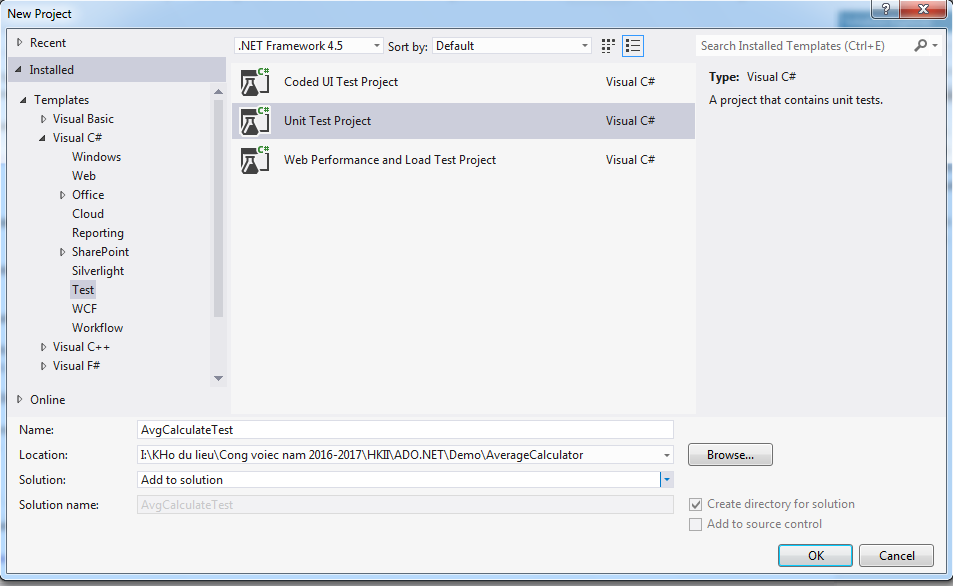• The AvgCalculateTest project is added to the AverageCalculator solution.

• In the AvgCalculateTest, add a reference to the AverageCalculator solution by selecting References in the avgCalculateTest project in Solution Explorer and then choose Add Reference… from the context menu.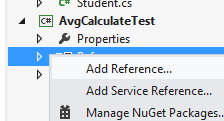• In the Reference Manager dialog box, expand Solution and then check AverageCalculator item.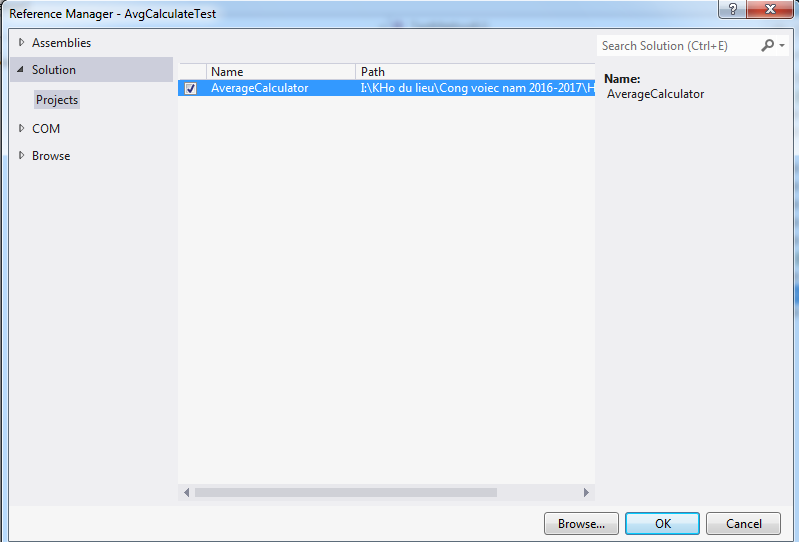• Click OK.

### Create the First Test Method

``````[TestMethod]
public void TestCityStudent()
{
double dbmath = 4.00;
double dbliteral = 3.00;
double expected = 3.5;
CityStudent st = new CityStudent();
st.MATH = dbmath;
st.LITERAL = dbliteral;
double actual = st.AvgCalculate();
Assert.AreEqual(expected,actual);
}``````

In the Build menu, choose Build Solution.

Choose Run All in the Test Explorer window: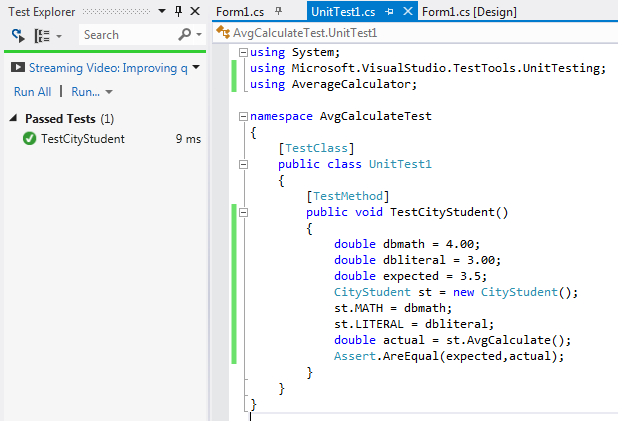### Create the Second Test Method

``````[TestMethod]
public void TestCountryStudent()
{
double dbmath = 4.00;
double dbliteral = 3.00;
double expected = 4.0;
CountryStudent st = new CountryStudent();
st.MATH = dbmath;
st.LITERAL = dbliteral;
double actual = st.AvgCalculate();
Assert.AreEqual(expected, actual);
}``````

Here is the result: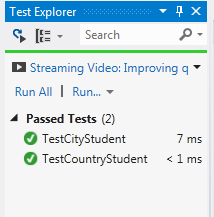In this article, I have introduced how to use refactoring and unit test in my C# code improving process. It is only a simple example but I hope that it is helpful for someone who are caring about code quality, refactoring, and unit testing.

Topics:
refactoring ,unit testing ,code quality ,tutorial ,devops

Comment (2)

Save
{{ articles.views | formatCount}} Views

Opinions expressed by DZone contributors are their own.